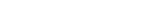MathExamle.com# Decimal

Select from the list a generator that creates examples with decimal answers and numbers

### Partitive

Creates examples with addition and subtraction operations of decimals, the answers are always positive

3 Variants 4 Levels
 72+22.88= 11.58+84.27= 81.69-52.51= 26.24-25.89=
Variant: 1 Level: Difficult

Creates examples with a division operation of decimals by a column method

1 Variant 3 Levels
 30.6 8.5
 15.4 7
 1.1 0.2
 46.2 2
Variant: 1 Level: Easy

Creates examples with multiplication and division of decimals

5 Variants 4 Levels
 97.2÷1.5= 63.8÷2.2= 36÷0.6= 28.5÷1.5= 16.4÷0.5= 31.6÷4=
Variant: 2 Level: Easy

Creates expressions with the operation of rounding decimals

3 Variants 3 Levels
 3.63+27.94≈ 65.17≈ 61.68×1.4≈ 21.52≈
Variant: A Level: Normal

Creates expressions with comparisons of decimals, the numbers are always positive

3 Variants 4 Levels
 34+26.2 = 8.1+52.1 18.6+45 > 14.9+42.9
Variant: 2 Level: Easy

Creates examples with a long division of decimals according to the British system

1 Variant 3 Levels
 33 1.32
 0.46 24.472
 0.032 0.028
Variant: 1 Level: Difficult

Creates examples with addition and subtraction operations of decimals, the answers can be negative

3 Variants 4 Levels
 3.98-(-13.02-57)= -47+23+77.37= 21-21.29-73.7= 12+46=
Variant: A Level: Difficult

Creates examples with a division operation of integers, the answers may contain a decimal point

1 Variant 3 Levels
 16÷40= 58÷40= 74÷8= 99÷12= 76÷8= 20÷5=
Variant: 1 Level: Normal

Creates examples with operations of addition, subtraction, multiplication and division of decimals, the answers are always positive

3 Variants 4 Levels
 89.87+20×0.38= 20-8×0.8÷1.6= 30-0.5×2.4+25.46= 14×3.61+10.8÷90=
Variant: A Level: Normal

Creates examples with operations of addition, subtraction, multiplication and division of decimals, the answers can be negative

3 Variants 4 Levels
 30.4-8.16÷0.4= -33.26-52.92÷18.9= -5.44-10.25×0.64= 15.84×2+30=
Variant: 1 Level: Normal

Creates expressions with comparisons of decimals, the numbers can be negative

3 Variants 4 Levels
 -12.34-57.66 < 32.82+37.17 -54.66 < 6
Variant: A Level: Normal

Creates examples with a long division of integers according to the British system, the answers may contain a decimal point

1 Variant 3 Levels
 23÷50= 5÷50= 12÷15= 54÷50= 21÷10= 100÷25=
Variant: 1 Level: Normal